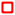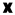Number MasterThe object of the game is to guess the hidden four digit number. There are no repeated digits in the number. Once you have guessed, a code will be shown that indicates how successful your guess was.

Enter your four digit guess below:= correct digit in the right place= correct digit in the wrong place= incorrect digit [These do not tell you the correct position of any digit]Example GameIf the number was 8695 then random guesses would reveal (starting at the bottom):

 10 5968= four correct, wrong place 9 8652= two correct, correct place + one correct, wrong place 8 7421= none correct 7 7512= one correct, wrong place 6 9510= two correct, wrong place 5 5217= one correct, wrong place 4 0325= one correct, correct place 3 9652= one correct, correct place + two correct, wrong place 2 5678= one correct, correct place + two correct, wrong place 1 1234= none correct